# costume ()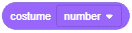## Description

The block holds its sprite’s current costume number.

## Example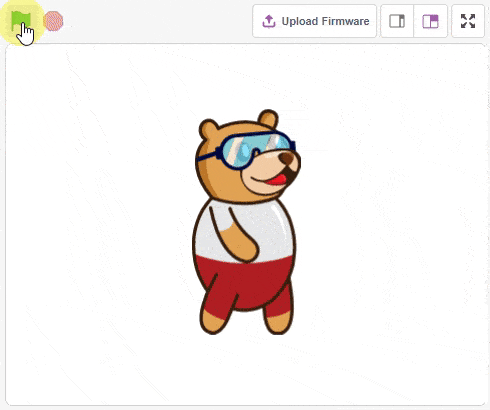### Costumes in PictoBlox

The example demonstrates the costume change in PictoBlox.

#### Script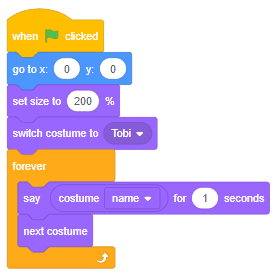#### Output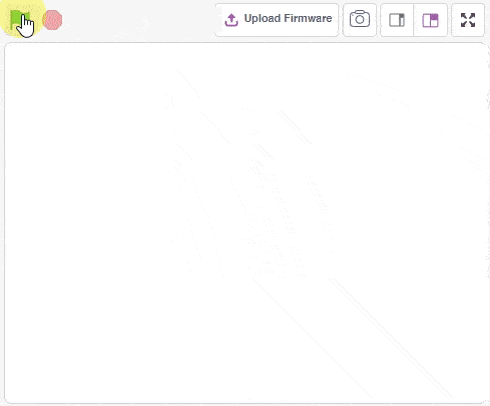### Covid Experiment – Velocity

In this example, we simulate the covid experiment using the physics simulation.

In this example, we simulate the covid experiment using the physics simulation. Following is what we are doing:

1. 21 humans are created with 20 green and 1 red ball.
2. Gravity is set to 0 simulating zero gravity.
3. The balls move at random speeds constantly creating random behavior.
4. If the green balls touch the red balls, it turns red.
5. After 10 seconds the red ball gets converted to green.

#### Script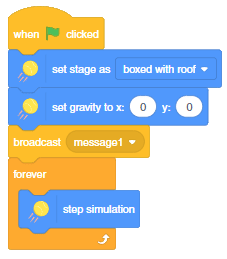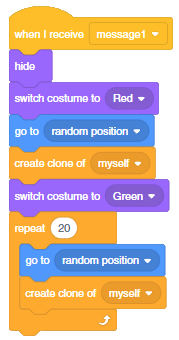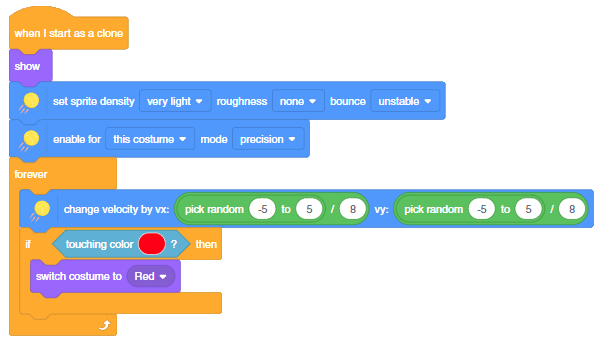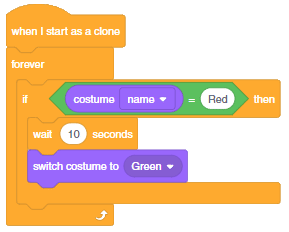#### Output